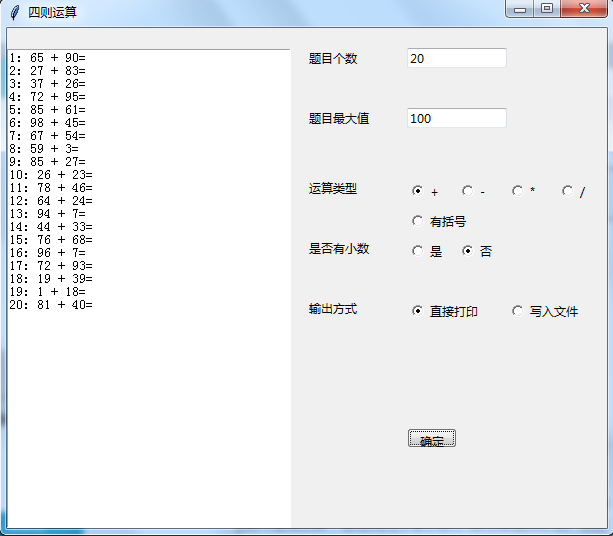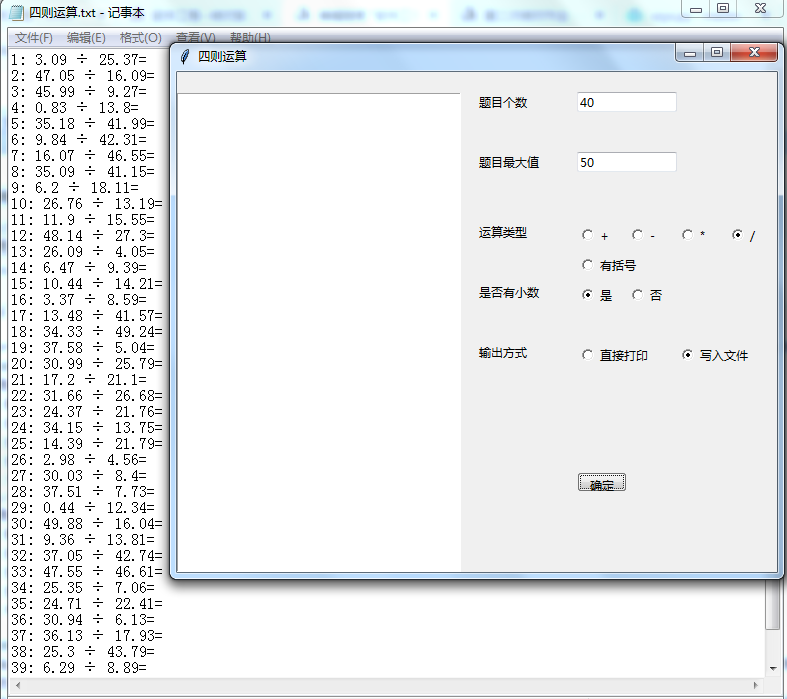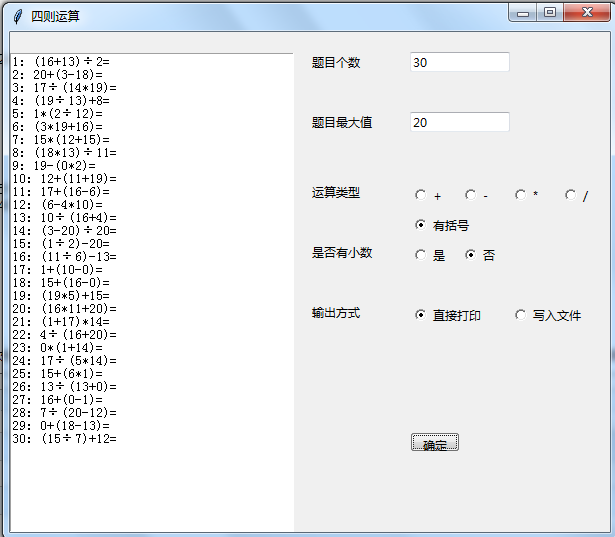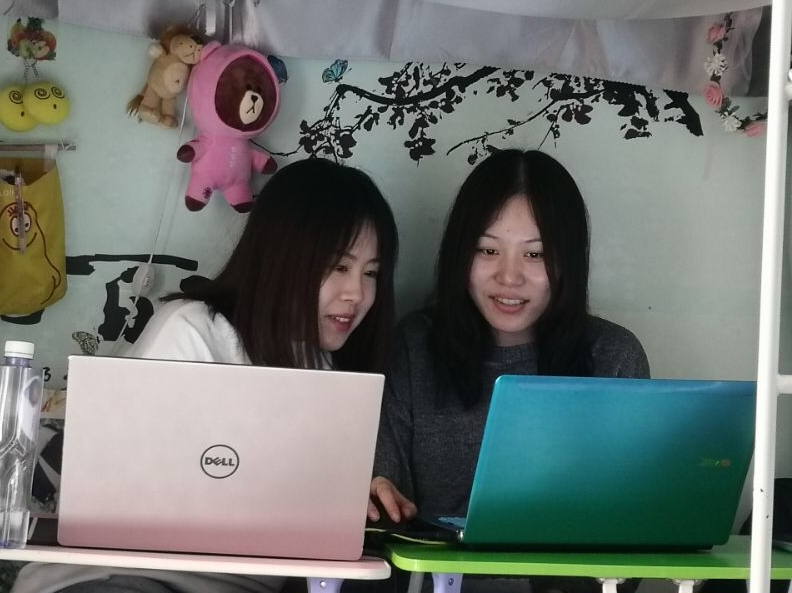# 软件工程（2018）结对编程第二次作业

(1)能够自动生成四则运算练习题
(2)可以定制题目数量
(3)用户可以选择运算符
(4)用户设置最大数（如十以内、百以内等）
(5)用户选择是否有括号、是否有小数
(6)用户选择输出方式（如输出到文件、打印机等）
(7)最好能提供图形用户界面（根据自己能力选做，以完成上述功能为主）

<font size="3"color="green">驾驶员---于秀---<a style="text-decoration:none;color:orange"href="https://www.cnblogs.com/yuxiu-/">我的博客

(1)能够完成全部代码工作，程序基本实现全部要求功能，并将代码上传至coding.net或者GitHub代码托管系统中
(2)能够对导航员在本次编程工作中起到的作用给出客观评价，并完成500字以上的总结，并且上传和队友工作时的照片
<font size="3"color="green">领航员---王雅婷---<a style="text-decoration:none;color:orange"href="https://www.cnblogs.com/Ww-Yating/">王雅婷的博客

(1)能够辅助驾驶员完成全部代码工作，并且为关键函数选用合适的覆盖标准设计测试用例，并编写代码进行单元自动测试
(2)能够根据上次作业的代码检查表对驾驶员的本次工作进行评价
(3)能够对本次工作完成500字以上的总结
<a style="text-decoration:none;color:orange"href="https://dev.tencent.com/u/Ww-Yating/p/sizeyunsuan">coding 戳我查看测试代码

1.图形化界面设计代码
使用了两个输入框进行题目个数和题目最大值的值，采用单选框实现运算符、是否有小数、以及输出方式的选择，利用文本框展示选择直接打印的结果。

lb = Label(root)
lb.pack()
text = Text(root,x=0,y=0, width=40, height=100)
text.pack(anchor=W)

Lab1=Label(root,text='题目个数').place(x=300,y=20)
default_value = StringVar()
default_value.set('1')
inp1 = Entry(root,textvariable = default_value)
inp1.place(x=400, y=20, width=100, height=20)

Lab2=Label(root,text='题目最大值').place(x=300,y=80)
default_value1 = StringVar()
default_value1.set('10')
inp2 = Entry(root,textvariable=default_value1)
inp2.place(x=400, y=80, width=100, height=20)

Lab3=Label(root,text='运算类型').place(x=300,y=150)
var=tk.IntVar()

Lab3=Label(root,text='是否有小数').place(x=300,y=210)
var1 = tk.IntVar()

Lab4=Label(root,text='输出方式').place(x=300,y=270)
var2 = tk.IntVar()

btn1 = Button(root, text='确定', command=Mysel)
btn1.place(x=400, y=400, width=50, height=20)


2.四则运算的加法函数
首先生成两个随机数，根据是否有小数的标志位决定随机数是整数还是小数，将生成的随机数和加号组成一个加法表达式存在字符串中，根据选择的存入文件还是直接打印进行相应的输出。

def add(i):
if flag==0:
a=random.randint(0,n)
b=random.randint(0,n)
else:
a=random.uniform(0,n)
a=round(a,2)
b=random.uniform(0,n)
b=round(b,2)
global s
s=str(i+1)+": "+str(a)+" + "+str(b)+"="
s1=round(a+b,2)
if flag1==0:
s=s+'\n'
text.insert(INSERT, s)
else:
f=open("四则运算.txt","a+")
f.write(s)
f.write("\n")
f.close()
return a+b


3.混合运算
由于生成简单的四则运算，所以混合运算采用三个随机数以及括号组成。随机生成三个整数或者三个小数，随机生成两个运算符以及一对括号，组成一个算术表达式存在字符串中，根据选择的存入文件还是直接打印进行相应的输出。

def mix(i):
op=['+','-','*','÷']
if flag==0:
a=random.randint(0,n)
b=random.randint(0,n)
c=random.randint(0,n)
else:
a=random.uniform(0,n)
a=round(a,2)
b=random.uniform(0,n)
b=round(b,2)
c = random.uniform(0, n)
c = round(c, 2)
ch1=random.randint(0,1)
ch2=random.randint(1,2)
if ch1==ch2:
s=str(i+1)+": "+str(a)+str(op[random.randint(0,3)])+"("+str(b)+
str(op[random.randint(0,3)])+str(c)+")"+"="+"\n"
if ch1==0 and ch2==1:
s=str(i+1)+": "+"("+str(a)+str(op[random.randint(0, 3)])+str(b)+")"+
str(op[random.randint(0, 3)])+str(c)+"="+"\n"
if ch1==0 and ch2==2:
s=str(i+1)+": "+"("+str(a)+str(op[random.randint(0, 3)])+str(b)+
str(op[random.randint(0, 3)])+str(c)+")"+"="+"\n"
if ch1==1 and ch2==2:
s=str(i+1)+": "+str(a)+str(op[random.randint(0, 3)])+"("+str(b)+
str(op[random.randint(0, 3)])+str(c)+")"+"="+"\n"
if flag1==0:
text.insert(INSERT, s)
if flag1==1:
f=open("四则运算.txt","a+")
f.write(s)
f.close()
return


<a style="text-decoration:none;color:orange"href="https://dev.tencent.com/u/xiu_er/p/sizeyunsuan/git/blob/master/szys.py">coding 戳我查看代码

1.加法测试
（1）数值范围在100以内
（2）选择加法算符
（3）生成20道题
（4）生成的题目直接打印
（5）无小数2.除法测试
（1）数值范围在50以内
（2）选择除法算符
（3）生成40道题
（4）生成的题目写入文件
（5）有小数3.混合测试
（1）数值范围在20以内
（2）选择有括号
（3）生成30道题
（4）生成的题目直接打印
（5）无小数本次结对作业是第一次和我的同伴在一起进行结对编程，在这次结对作业中，我扮演的是驾驶员的角色，我的同伴王雅婷同学扮演的是领航员的角色，我们之间合作非常愉快。
首先，我的同伴是一个比较细心的人，所以在这次编编写代码过程中，我的同伴能够很准确及时地发现我犯下的小错误，在我身边督促我，避免了更大错误的发生。其实我觉得结对编程并不是严格意义上的一个人编写代码，另一个人在看，而是两个人在共同讨论出思路后，驾驶员能够很快的将思路整理成代码，而领航员也能够负责检查，这一点上，我的同伴做的很好，在我编写代码的过程中，她能够和我一起查找必要的资料理清思路，这让我充分意识到了结对编程的好处。总的来说，我的同伴很好的完成了领航员的角色。

在本次结对编程过程中，我扮演的是驾驶员的角色，虽然这是第一次真正意义上的结对编程，但我们完成的比较顺利。这次驾驶员的角色的确让我发现了自己在编程过程中不好的习惯，但能够及时发现才是最大的收获。
我认为驾驶员应当在编程过程中起到带头作用，应当及时理清思路编写代码，还应该积极听取同伴的建议，遇到问题要学会分享，与同伴分享的过程也是在理清自己的思路。这次的结对编程作业相比于上一次有一定的难度，但总体上还是完成的比较圆满，这次作业让我意识到了合作的重要性和不同角色的定位和功能，比如作为驾驶员在面对相对复杂的算法时，能够有领航员自始至终跟上自己的思路，及时找出编程中的漏洞并提出具有建设性的建议是一件非常有意义的事，这提高了编程的效率与准确性，并且我的同伴还能在没有思路时提出一个好的想法，这就是结对编程的意义，这也让我更加期待之后的团队项目。
当然了，这次的结对编程作业给我带来的最大的收获还是人多力量大，让我充分理解了什么是1+1>2。总体上来说，虽然过程中我们遇到了不少比较棘手的问题，但最终还是能够基本完成四则运算题目的基本要求，我也通过这次的结对编程意识到了自己的不足，希望在日后的编程过程中能够有所改进。希望以后还会有机会和王雅婷同学一起交流学习。posted @ 2019-05-06 15:02  于秀  阅读(209)  评论(0编辑  收藏  举报